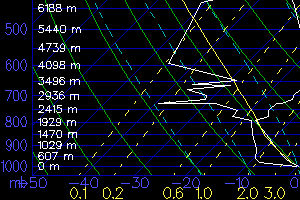Search:

Upper Air Data

In this section, you will find many of the upper air images that Meteorologists use to make detailed forecasts. These images come from Unisys. In addition, you will find various indicies that a meteorologist could use to aid in the forecasting. When you see "MB" listed for a particular plot, it stands for millibars. If you don't know exactly what a millibar is, click here for a definition. Each plot shows a different level of the atmosphere. There are several sounding data sets available, including skew-T diagrams, hodographs, and stuve.Above: Skew-T Diagram

[ Sounding Data | Severe Weather Contours | 925 MB Plots | 850 MB Plots | 700 MB Plots ]
[ 500 MB Plots | 400 MB Plots | 300 MB Plots | 250 MB Plots ]
[ 200 MB Plots | 150 MB Plots | 100 MB Plots ]

 Sounding Data Skew-T/Hodograph/Stuve/TextSevere Weather Contours

 925 MB Plots

 850 MB Plots

 700 MB Plots

 500 MB Plots

 400 MB Plots

 300 MB Plots

 250 MB Plots

 200 MB Plots

 150 MB Plots

 100 MB Plots